## Excel BINOM.DIST.RANGE Function

The BINOM.DIST.RANGE function calculates the binomial distribution probability for the number of successes from a specified number of trials falling into a specified range.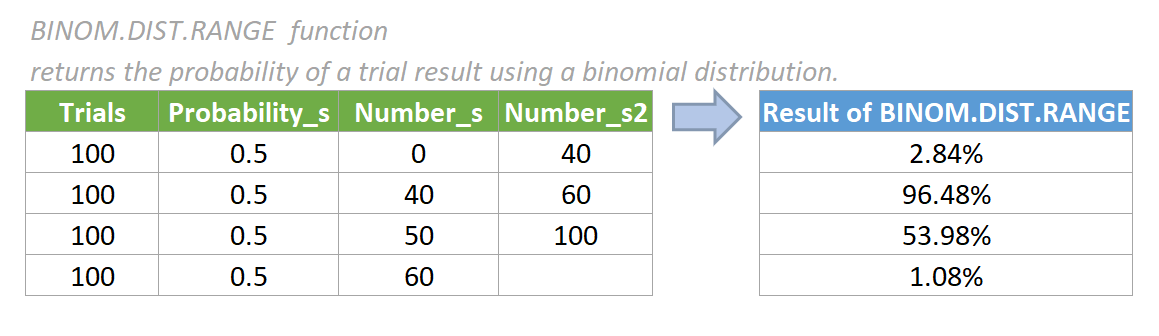#### Syntax

=BINOM.DIST.RANGE(trials,probability_s,number_s,[number_s2])

#### Arguments

• Trials (required): The number of independent trials. It must be greater than or equal to 0.
• Probability_s (required): The probability of success in each trial. It must be greater than or equal to 0 and less than or equal to 1.
• Number_s (required): The number of successes in trials. It must be greater than or equal to 0 and less than or equal to the number of trials.
• Number_s2 (optional): The upper value for a number of successes. When provided, BINOM.DIST.RANGE returns the probability that the number of successful trials is between number_s and number_s2. It must be greater than or equal to number_s and less than or equal to the number of trials.

#### Return value

The BINOM.DIST.RANGE function returns a numeric value.

#### Function notes

1. The BINOM.DIST.RANGE function truncates all numerical arguments except probability_s to integer.
2. The #VALUE! error value occurs when any of the arguments is non-numeric.
3. The #NUM! error value occurs if:
• the supplied trials argument is < 0
• the supplied probability_s argument is < 0 or > 1
• the supplied number_s argument is < 0 or > trials
• the supplied number_s2 argument is < 0 or > trials or < number_s
4. The BINOM.DIST.RANGE function is newly introduced in Excel 2013, so it is not available in earlier versions of Excel.
5. The equation of BINOM.DIST.RANGE function is: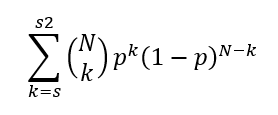In the equation above, N represents Trials, p represents Probability_s, s represents Number_s, s2 represents Number_s2, and k represents the iteration variable.

#### Examples

In this case, we want to calculate the binomial distribution probability based on the data provided in the table below, please do as follows.

1. Please copy the formula below into cell G5, then press the Enter key to get the result.

=BINOM.DIST.RANGE(B5,C5,D5,E5)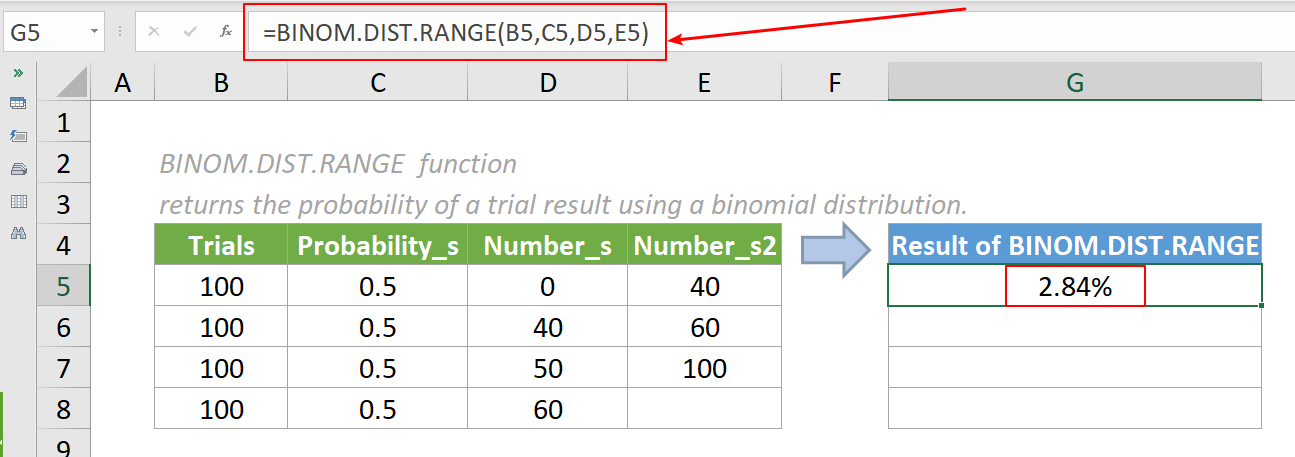2. Select this result cell and drag its autofill handle down to get the rest of the results.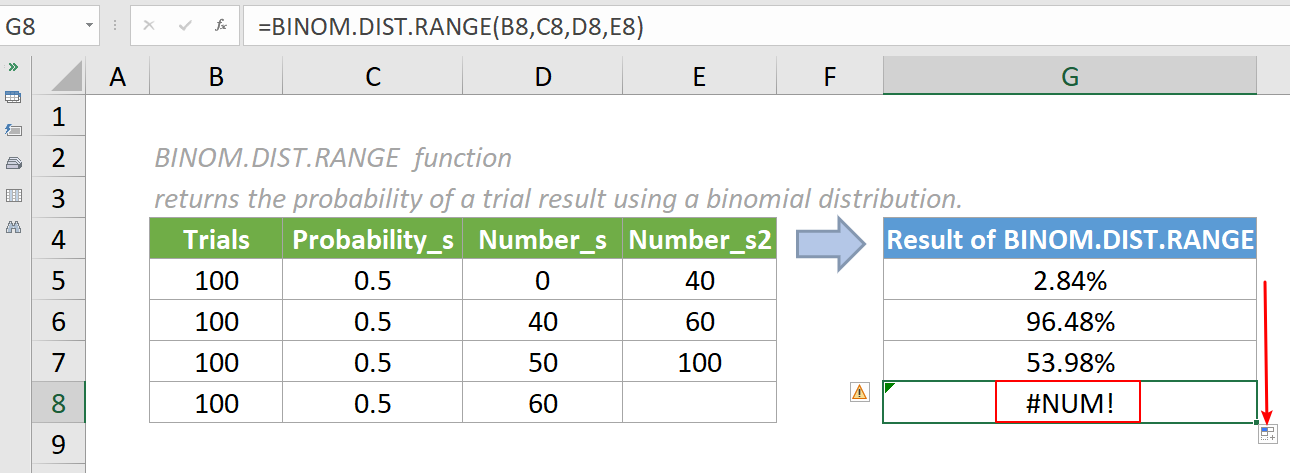3. The last result is a #NUM! error because E8, the cell of optional number_s2 argument, shouldn’t be included in the formula. The cell reference is empty and is treated as 0 value in the formula, leading to a #NUM! error. The formula in G8 should be changed to:

=BINOM.DIST.RANGE(B8,C8,D8)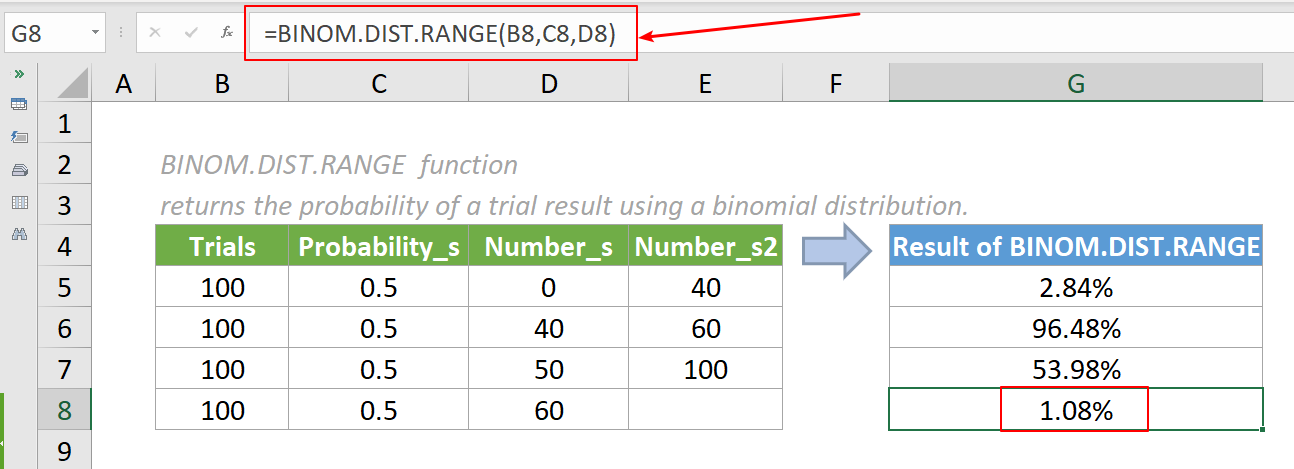Notes:

1. By default, the results are displayed as numbers. You can change the format of the result cells to percentage before or after applying the formulas.
2. In cell G5, the formula returns the binomial distribution probability that the number of successful trials is between 0 and 40.
3. In cell G8, the formula returns the binomial distribution probability that the number of successful trials is exactly 60.
4. We can also directly input a value in the formula. For example, the formula in cell G5 can be changed to:

=BINOM.DIST.RANGE(100,0.5,0,40)

#### Relative Functions:

• Excel EVEN Function
The EVEN function rounds numbers away from zero to the nearest even integer.

• Excel EXP Function
The EXP function returns the result of the constant e raised to the nth power.

### The Best Office Productivity Tools

#### Kutools for Excel - Helps You To Stand Out From Crowd

Would you like to complete your daily work quickly and perfectly? Kutools for Excel brings 300 powerful advanced features (Combine workbooks, sum by color, split cell contents, convert date, and so on...) and save 80% time for you.

• Designed for 1500 work scenarios, helps you solve 80% Excel problems.
• Reduce thousands of keyboard and mouse clicks every day, relieve your tired eyes and hands.
• Become an Excel expert in 3 minutes. No longer need to remember any painful formulas and VBA codes.
• 30-day unlimited free trial. 60-day money back guarantee. Free upgrade and support for 2 years.#### Office Tab - Enable Tabbed Reading and Editing in Microsoft Office (include Excel)

• One second to switch between dozens of open documents!
• Reduce hundreds of mouse clicks for you every day, say goodbye to mouse hand.
• Increases your productivity by 50% when viewing and editing multiple documents.
• Brings Efficient Tabs to Office (include Excel), Just Like Chrome, Firefox, And New Internet Explorer.